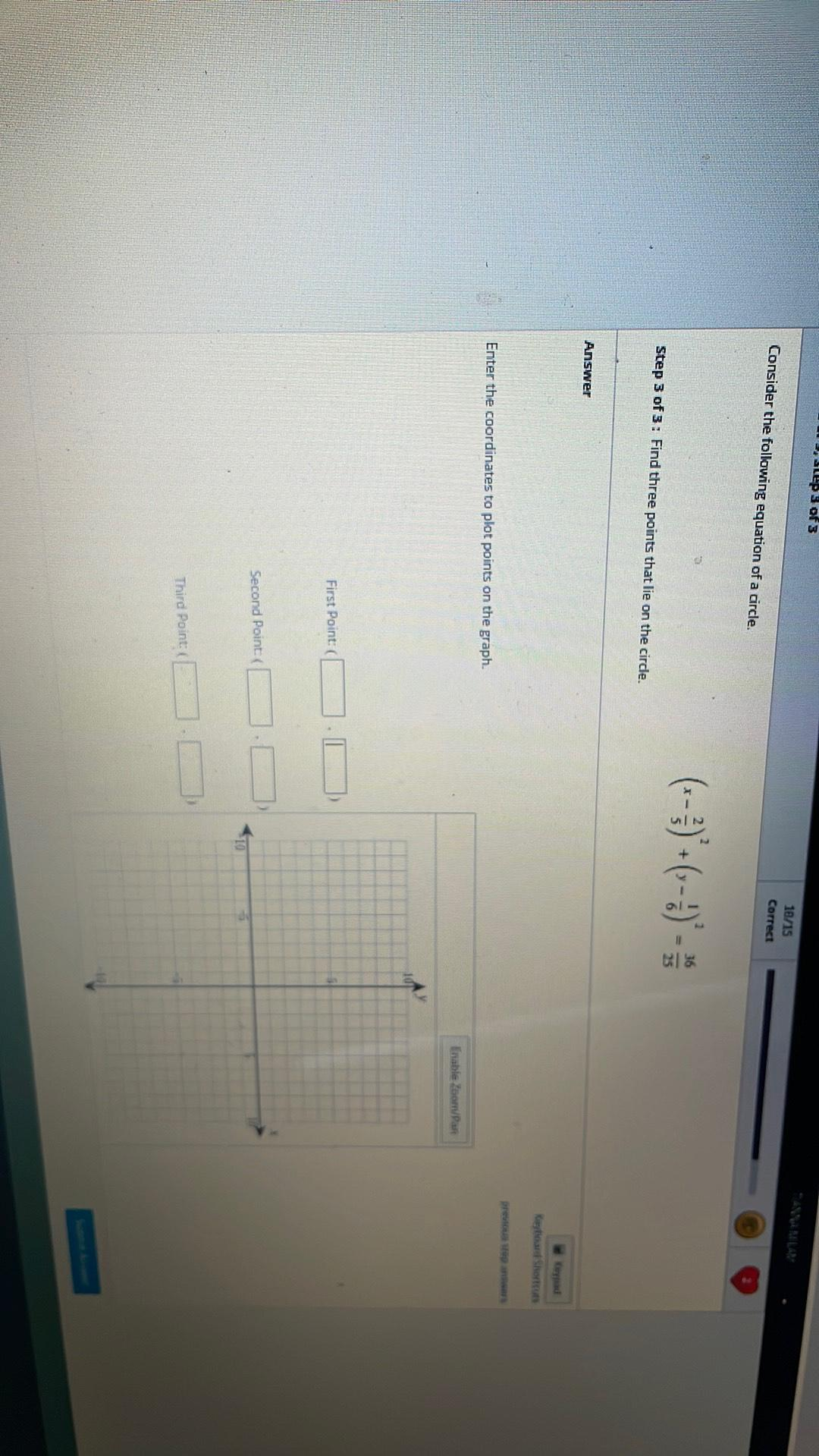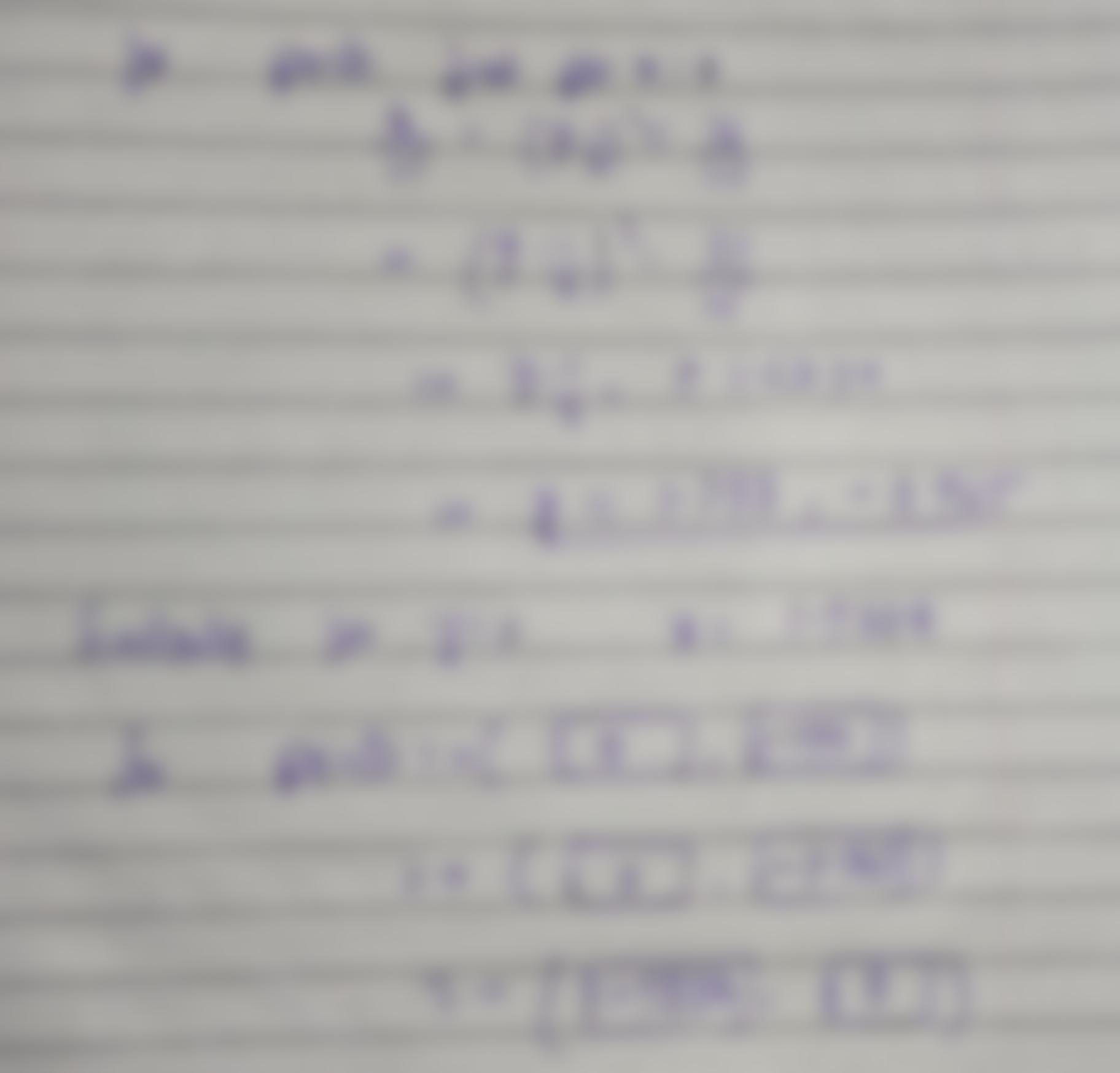Question:

SLEPs of 3 Consider the following equation of a circle. 10/15 Correct 36 25 Step 3 of 3: Find three points that lie on the circlSLEPs of 3 Consider the following equation of a circle. 10/15 Correct 36 25 Step 3 of 3: Find three points that lie on the circle. Answer res Enter the coordinates to plot points on the graph. Enable Zoomar First Point: Second Point 10 Third Point: